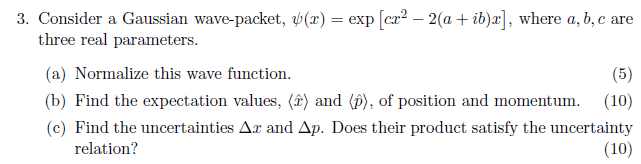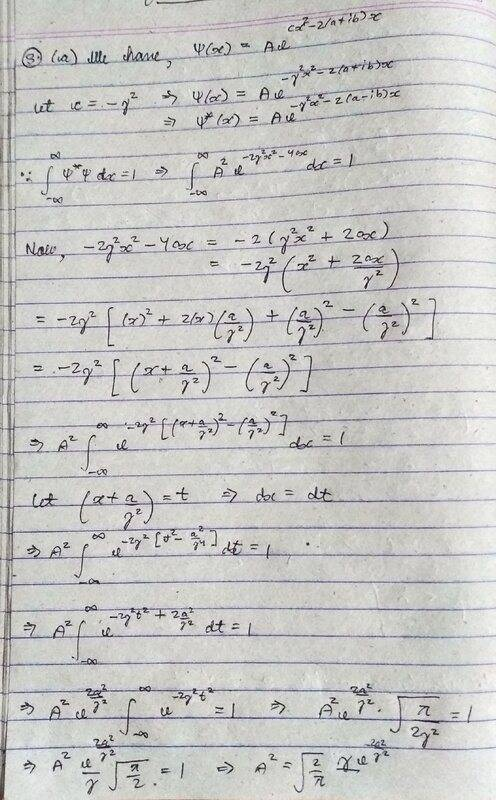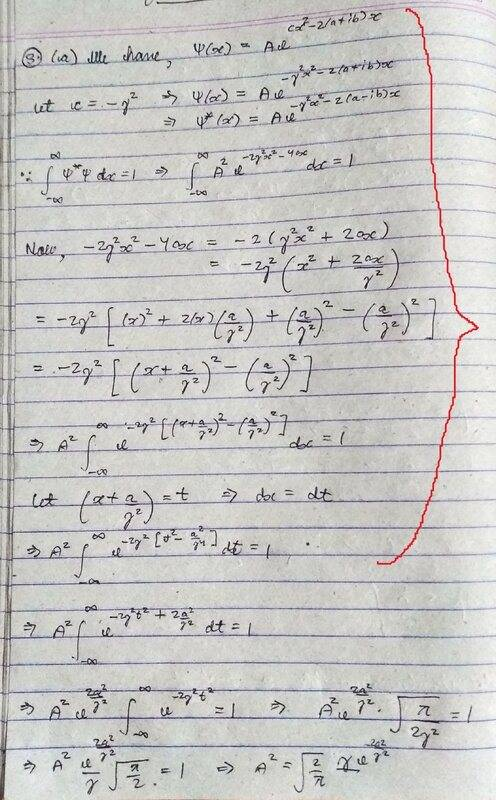# Normalize the Gaussian wave packet

tanaygupta2000
Homework Statement:
To normalize the wave equation given
Relevant Equations:
A^2 (psi)*(psi) = 1For normalizing this wave function, I began by finding the complex conjugate of psi and then multiplied it with the original psi.
Now what I am getting is A^2 integral exp(2cx^2-4ax) dx = 1
Now I am not getting how to solve this exponential term. I tried by completing the square method but it is not giving a good result. Please help!

Homework Helper
Gold Member
I would try completing the square of the exponential argument before multiplying by the complex conjugate and then do a change of variables. Why is it not a good result?

On edit: I take it back. Completing the square after finding ##\psi^*\psi## works. Note that given real constant ##c## must be negative for the normalization integral to converge and needs a little bit of extra care.

Last edited:
•tanaygupta2000
tanaygupta2000
Yes sir, integral will only converge if c is replaced by -c.
On doing so, and making some simple substitution like (x + a/c) = t and dx = dt,
I am successfully getting value of normalization constant A = (2c/pi)^(1/4) exp(-a^2 /c)

Homework Helper
Gold Member
I would write the coefficient up front as ##\left[\frac{2(-c)}{\pi}\right]^{\frac{1}{4}}.## That's because ##c## is a negative number. Also, the argument of the exponential is not what I got. What does your completed square for ##\psi^*\psi## look like?

tanaygupta2000
It's 2c[(x - a/c)^2 - (a/c)^2]

Homework Helper
Gold Member
It's 2c[(x - a/c)^2 - (a/c)^2]
Yes, I agree. Your normalization is good. Sorry for the confusion, I had an extra factor of two. Can you finish the problem now?

•tanaygupta2000
tanaygupta2000
Yes, I agree. Your normalization is good. Sorry for the confusion, I had an extra factor of two. Can you finish the problem now?
Sure, sir. Thank you very much for the help!
So I get this: solve by using c --> -c so that the integral can be evaluated and in the final answer revert it to +c back (using iota wherever necessary).

Homework Helper
Gold Member
Sure, sir. Thank you very much for the help!
So I get this: solve by using c --> -c so that the integral can be evaluated and in the final answer revert it to +c back (using iota wherever necessary).

I think you missed my point in post #4. You do not use ##i=\sqrt{-1}## wherever necessary. To normalize, you do the integral $$1=\int (N\psi)^*(N\psi) dx=|N|^2 \int \psi^*\psi~dx$$ and solve for ##|N|.## You can drop the absolute signs, but you must keep in mind that the normalization constant ##N## is real. To remember that in this case, it helps to write the constant up front as ##k=\left[\frac{2(-c)}{\pi}\right]^{\frac{1}{4}}.## If someone gives you a value ##c=-8~\text{m}^{-2}##, then you can substitute and say ##k=\frac{2}{\pi^{1/4}}~\text{m}^{-1/2}.## If someone gives you a value ##c=+8~\text{m}^{-2}##, then you cannot substitute and must say "this wavefunction cannot be normalized"; you don't say ##k=\frac{\sqrt{2}+i\sqrt{2}}{\pi^{1/4}}~\text{m}^{-1/2}.##

What did you get for the constant exponential term that multiplies ##k## when you write ##N=k \exp(?)##. I don't think it is ##\exp(-a^2/c^2)## as you have in post #3.

Last edited:
•tanaygupta2000
tanaygupta2000
Okay, so we write N = [-2c/pi]^(1/4) with c strictly negative as the normalization constant.
So for calculating the expectation values, can I use
psi(x) = [2c/pi]^(1/4) exp(-cx^2 - 2(a+ib)x - a^2 /c) ?

because for this integral to convege, there should be a - sign before the c.
So I replaced c with -c to evaluate it and hence there is no - sign in N.
Or should I adopt some other method?

Homework Helper
Gold Member
If you replace ##c## with ##-c##, you are likely to be confused in the end. What I would do is replace ##c=-\gamma^2## where ##\gamma## is real. Do your calculations and integrals with that and then in the very end replace ##\gamma^2## with ##(-c)## (keep the parentheses) and ##\gamma^4## with ##c^2##. That will automatically sort out the negative signs.

•tanaygupta2000
tanaygupta2000
In the end, I am getting a factor of sqrt(gamma) in the normalization constant.
How to justify it?

Homework Helper
Gold Member
In the end, I am getting a factor of sqrt(gamma) in the normalization constant.
How to justify it?
I don't get a factor of gamma. Please show me how it comes about in your calculation.

Last edited:
tanaygupta2000Homework Helper
Gold Member
You have ##A^2e^{2a^2/\gamma^2}\sqrt{\frac{\pi}{2\gamma^2}}=1##.
If you follow the algebra, it should be ##A^2e^{2a^2/\gamma^4}\sqrt{\frac{\pi}{2\gamma^2}}=1##.

Then ##A^2=e^{-2a^2/\gamma^4}\left[\frac{2\gamma^2}{\pi}\right]^{1/2}=e^{-2a^2/c^2}\left[\frac{2(-c)}{\pi}\right]^{1/2}.##

•tanaygupta2000
tanaygupta2000Sir, am I correct upto this portion?
After this step, I am getting only (gamma)^2 below 2a^2 as (gamma)^2/ (gamma)^4 = (gamma)^(-2). isn't it?

Homework Helper
Gold Member
2022 Award
View attachment 278261

Sir, am I correct upto this portion?
After this step, I am getting only (gamma)^2 below 2a^2 as (gamma)^2/ (gamma)^4 = (gamma)^(-2). isn't it?
That looks right to me.

Homework Helper
Gold Member
2022 Award
Here's an alternative approach I would take to break the problem down into easier steps:

First, evaluate: $$\int_{-\infty}^{+\infty} e^{-x^2 -\beta x}dx$$ And write down this answer and keep it somewhere safe.

Then, using this result, evaluate $$\int_{-\infty}^{+\infty} e^{-\alpha x^2 -\beta x}dx$$ using the substitution ##u = \sqrt{\alpha} x##. And keep that safe too.

(I have six pages of standard integrals like this! I find it faster and more reliable than typing things into Wolfram.)

Finally, evaluate your integral with ##\alpha = -2c, \ \beta = 4a##.

You can figure out ##A## at the end.

•tanaygupta2000
Homework Helper
Gold Member
2022 Award
You have ##A^2e^{2a^2/\gamma^2}\sqrt{\frac{\pi}{2\gamma^2}}=1##.
If you follow the algebra, it should be ##A^2e^{2a^2/\gamma^4}\sqrt{\frac{\pi}{2\gamma^2}}=1##.

Then ##A^2=e^{-2a^2/\gamma^4}\left[\frac{2\gamma^2}{\pi}\right]^{1/2}=e^{-2a^2/c^2}\left[\frac{2(-c)}{\pi}\right]^{1/2}.##
I get no more than ##\gamma^2## in there. And I'm just looking up my standard integrals!

tanaygupta2000
Here's an alternative approach I would take to break the problem down into easier steps:

First, evaluate: $$\int_{-\infty}^{+\infty} e^{-x^2 -\beta x}dx$$ And write down this answer and keep it somewhere safe.

Then, using this result, evaluate $$\int_{-\infty}^{+\infty} e^{-\alpha x^2 -\beta x}dx$$ using the substitution ##u = \sqrt{\alpha} x##. And keep that safe too.

(I have six pages of standard integrals like this! I find it faster and more reliable than typing things into Wolfram.)

Finally, evaluate your integral with ##\alpha = -2c, \ \beta = 4a##.

You can figure out ##A## at the end.
Thank You sir! But unfortunately the normalization constant is still coming imaginary.

tanaygupta2000
I'm getting confused.

Homework Helper
Gold Member
2022 Award
Thank You sir! But unfortunately the normalization constant is still coming imaginary.
Your answer for ##A^2## is real and positive in post #15. That is correct.

All these integrals are real and positive. They can't possibly have a negative value. The only confusion is that they gave you ##c## (which forces ##c## to be a negative number). Or, let ##c = -\gamma^2##, where ##\gamma## is positive, as you've done.

•tanaygupta2000
tanaygupta2000
Your answer for ##A^2## is real and positive in post #15. That is correct.

All these integrals are real and positive. They can't possibly have a negative value. The only confusion is that they gave you ##c## (which forces ##c## to be a negative number). Or, let ##c = -\gamma^2##, where ##\gamma## is positive, as you've done.
But my value of A is coming to be complex. It must be real.

Homework Helper
Gold Member
2022 Award
But my value of A is coming to be complex. It must be real.
Why is ##A## complex?

tanaygupta2000
Why is ##A## complex?
It is involving sqrt of iota.

tanaygupta2000
after making substitution c = -(gamma)^2

Homework Helper
Gold Member
2022 Award
It is involving sqrt of iota.
Not in post #15, where you have the right answer.

tanaygupta2000
Not in post #15, where you have the right answer.
Gamma = iota times c
So already-complex A will be more complex as sq root of i comes into picture.
I am wondering is the question incorrect?

Homework Helper
Gold Member
2022 Award
Gamma = iota times c
So already-complex A will be more complex as sq root of i comes into picture.
I am wondering is the question incorrect?
No, no, no!

##c## is negative, ##-c## is positive, ##\gamma## is positive by definition.

There are no complex numbers involved.

•tanaygupta2000
tanaygupta2000
No, no, no!

##c## is negative, ##-c## is positive, ##\gamma## is positive by definition.

There are no complex numbers involved.
Excellent sir! Now I understood everything !
Thank You so so much for yours and Kuruman sir's precious efforts in helping me.

•kuruman and PeroK
Homework Helper
Gold Member
I get no more than ##\gamma^2## in there. And I'm just looking up my standard integrals!
You are absolutely correct. I forgot the ##\gamma^2## up front when I separated the exponentials. This is especially infuriating to me because I squared ##\gamma## in the first place so that its dimensions match ##a## and make troubleshooting easier by dimensional analysis.
##c## is negative,##-c## is positive, ##\gamma## is positive by definition
Actually the definition is ##c=-\gamma^2## where ##\gamma## is real. ##\gamma## doesn't have to be positive.

To @tanaygupta2000 :
Your expression in post #15 ##A^2e^{2a^2/\gamma^2}\sqrt{\frac{\pi}{2\gamma^2}}=1## is correct. Sorry for the confusion.

•tanaygupta2000